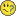# QlikView Layout & Visualizations

Discussion Board for collaboration on QlikView Layout & Visualizations.

Announcements
Save the Date: QlikWorld Online, June 24-25, 2020. Free global virtual event for data integration and data analytic gurus. Register TodayNot applicable

## Pie chart with no dimension

Hi,

I wonder if somebody can help me with a couple of expressions that are driving me mad.

I have a list of Clients to which I have assigned a certain Status (Green, Yellow and Red) that is calculated based on the user selections. For each Status I have a list box. Now I have to build a pie chart that shows how many Clients belong to each Status and another one that shows the sum of sales that was made to the Clients belonging to each Status. I guess my pie charts will need three expressions each and no dimensions but I don't seem to be able to make it work.

Below are the expressions that I've used to build each of the three list boxes.

GREEN:

aggr(

if(count(distinct Partner)=1

or

count(distinct Partner)=2

AND

count(distinct Year)>1

AND

only(aggr(if(rank(aggr(sum({<Year= {\$(=Max(Year)-2)}>} Sales),Client,Partner))=1,Partner),Client,Partner))

=

only(aggr(if(rank(aggr(sum({<Year= {\$(=Max(Year)-1)}>} Sales),Client,Partner))=1,Partner),Client,Partner))

or

count(distinct Partner)=2

AND

count(distinct Year)>1

AND

only(aggr(if(rank(aggr(sum({<Year= {\$(=Max(Year)-2)}>} Sales),Client,Partner))=1,Partner),Client,Partner))

=

if(sum({<Year={\$(=Max(Year))}>}Sales)>0, aggr(if(rank(aggr(sum({<Year={\$(=Max(Year))}>} Sales),\$(Variable1)))=1,Partner),\$(Variable1)), aggr(if(rank(aggr(sum({<Year={\$(=Max(Year)-1)}>} Sales),\$(Variable1)))=1,Partner),\$(Variable1)))

or

count(distinct Partner)=2

AND

count(distinct Year)>1

AND

only(aggr(if(rank(aggr(sum({<Year= {\$(=Max(Year)-1)}>} Sales),Client,Partner))=1,Partner),Client,Partner))

=

if(sum({<Year={\$(=Max(Year))}>}Sales)>0, aggr(if(rank(aggr(sum({<Year={\$(=Max(Year))}>} Sales),\$(Variable1)))=1,Partner),\$(Variable1)), aggr(if(rank(aggr(sum({<Year={\$(=Max(Year)-1)}>} Sales),\$(Variable1)))=1,Partner),\$(Variable1))),

Client), Client)

YELLOW:

aggr(

if( count(distinct Partner)>2

or

count(distinct Partner)=2

AND

only(aggr(if(rank(aggr(sum({<Year= {\$(=Max(Year)-2)}>} Sales),Client,Partner))=1,Partner),Client,Partner))

<>

only(aggr(if(rank(aggr(sum({<Year= {\$(=Max(Year)-1)}>} Sales),Client,Partner))=1,Partner),Client,Partner))

AND

only(aggr(if(rank(aggr(sum({<Year= {\$(=Max(Year)-2)}>} Sales),Client,Partner))=1,Partner),Client,Partner))

<>

if(sum({<Year={\$(=Max(Year))}>}Sales)>0, aggr(if(rank(

aggr(sum({<Year={\$(=Max(Year))}>} Sales),\$(Variable1))

)=1,Partner),\$(Variable1)), aggr(if(rank(

aggr(sum({<Year={\$(=Max(Year)-1)}>} Sales),\$(Variable1))

)=1,Partner),\$(Variable1)))

AND

only(aggr(if(rank(aggr(sum({<Year= {\$(=Max(Year)-1)}>} Sales),Client,Partner))=1,Partner),Client,Partner))

<>

if(sum({<Year={\$(=Max(Year))}>}Sales)>0, aggr(if(rank(aggr(sum({<Year={\$(=Max(Year))}>} Sales),\$(Variable1)))=1,Partner),\$(Variable1)), aggr(if(rank(aggr(sum({<Year={\$(=Max(Year)-1)}>} Sales),\$(Variable1)))=1,Partner),\$(Variable1)))

or

count(distinct Partner)=2

AND

count(distinct Year)=1,

Client), Client)

RED:

aggr(if(count(Year)=0,  Client), Client)

Tags (3)
1 Solution

Accepted Solutions
HighlightedNot applicable

## Re: Pie chart with no dimension

I've finally managed to solve this!

The correct expressions are as follows:

Green:

sum(

{< Client= {"=sum(distinctPartner)=1"}> }

Opportunity)

+

//All 3

sum(

{< Client= {"=sum(distinctPartner)=2 and

sum(distinctYear)>1 and

only(aggr(if(rank(aggr(sum({<Year= {\$(=Max(Year)-1)}>} Sales),Client,Partner))=1,Partner),Client,Partner))=

only(aggr(if(rank(aggr(sum({<Year= {\$(=Max(Year)-2)}>} Sales),Client,Partner))=1,Partner),Client,Partner)) and

only(aggr(if(rank(aggr(sum({<Year= {\$(=Max(Year)-1)}>} Sales),Client,Partner))=1,Partner),Client,Partner))=

if(sum({<Year={\$(=Max(Year))}>}Sales)>0, aggr(if(rank(

aggr(sum({<Year={\$(=Max(Year))}>} Sales),\$(Variable1))

)=1,Partner),\$(Variable1)), aggr(if(rank(

aggr(sum({<Year={\$(=Max(Year)-1)}>} Sales),\$(Variable1))

)=1,Partner),\$(Variable1)))"}> }

Opportunity)

+

//2011=MostRecent and 2011<>2010

sum(

{< Client= {"=sum(distinctPartner)=2 and

sum(distinctYear)>1 and

only(aggr(if(rank(aggr(sum({<Year= {\$(=Max(Year)-1)}>} Sales),Client,Partner))=1,Partner),Client,Partner))=

if(sum({<Year={\$(=Max(Year))}>}Sales)>0, aggr(if(rank(

aggr(sum({<Year={\$(=Max(Year))}>} Sales),\$(Variable1))

)=1,Partner),\$(Variable1)), aggr(if(rank(

aggr(sum({<Year={\$(=Max(Year)-1)}>} Sales),\$(Variable1))

)=1,Partner),\$(Variable1))) and

only(aggr(if(rank(aggr(sum({<Year= {\$(=Max(Year)-1)}>} Sales),Client,Partner))=1,Partner),Client,Partner))<>

only(aggr(if(rank(aggr(sum({<Year= {\$(=Max(Year)-2)}>} Sales),Client,Partner))=1,Partner),Client,Partner)) "}> }

Opportunity)

+

//2011=2010 and 2011<>MostRecent

sum(

{< Client= {"=sum(distinctPartner)=2 and

sum(distinctYear)>1 and

only(aggr(if(rank(aggr(sum({<Year= {\$(=Max(Year)-1)}>} Sales),Client,Partner))=1,Partner),Client,Partner))=

only(aggr(if(rank(aggr(sum({<Year= {\$(=Max(Year)-2)}>} Sales),Client,Partner))=1,Partner),Client,Partner)) and

only(aggr(if(rank(aggr(sum({<Year= {\$(=Max(Year)-1)}>} Sales),Client,Partner))=1,Partner),Client,Partner))<>

if(sum({<Year={\$(=Max(Year))}>}Sales)>0, aggr(if(rank(

aggr(sum({<Year={\$(=Max(Year))}>} Sales),\$(Variable1))

)=1,Partner),\$(Variable1)), aggr(if(rank(

aggr(sum({<Year={\$(=Max(Year)-1)}>} Sales),\$(Variable1))

)=1,Partner),\$(Variable1))) "}> }

Opportunity)

+

//2010=MostRecent and 2010<>2011

sum(

{< Client= {"=sum(distinctPartner)=2 and

sum(distinctYear)>1 and

only(aggr(if(rank(aggr(sum({<Year= {\$(=Max(Year)-2)}>} Sales),Client,Partner))=1,Partner),Client,Partner))=

if(sum({<Year={\$(=Max(Year))}>}Sales)>0, aggr(if(rank(

aggr(sum({<Year={\$(=Max(Year))}>} Sales),\$(Variable1))

)=1,Partner),\$(Variable1)), aggr(if(rank(

aggr(sum({<Year={\$(=Max(Year)-1)}>} Sales),\$(Variable1))

)=1,Partner),\$(Variable1))) and

only(aggr(if(rank(aggr(sum({<Year= {\$(=Max(Year)-2)}>} Sales),Client,Partner))=1,Partner),Client,Partner))<>

only(aggr(if(rank(aggr(sum({<Year= {\$(=Max(Year)-1)}>} Sales),Client,Partner))=1,Partner),Client,Partner)) "}> }

Opportunity)

Red:

sum(

{< Client= {"=sum(distinctPartner)=0"}> } Opportunity)

Yellow:

sum(Opportunity) - Red - Green

Went the easier way with the Yellow. Have also created a distinctPartner field to avoid all those count(distinct).

19 Replies
HighlightedNot applicable

## Re: Pie chart with no dimension

post a sample file

it'll be easier

HighlightedNot applicable

## Re: Pie chart with no dimension

It's kind of complicated - I'll see what I can do.

HighlightedNot applicable

## Re: Pie chart with no dimension

Ok now with sample file attached above.

HighlightedNot applicable

## Re: Pie chart with no dimension

Hi,

I think I'm almost there but there is one expression that is not working - see attached sample file.

In the pie chart, it looks like only the first part of the Green expression is being calculated - the total should be \$218,918 however, for some reason, Client XX KMOUHGDSAUYE HKNTJKMU HLJMOTP / Mvchndz is being calculated in the Yellow slice.

I'm sure I'm missing something silly but I see double right now so can somebody help please?Thanks

HighlightedNot applicable

## Re: Pie chart with no dimension

...only(aggr(if(rank(aggr(sum({<Year= {\$(=Max(Year)-2)}>} Sales),Client,Partner))=1,Partner),Client,Partner))....

this part of expression looks wrong (am i right?)

there can't be if after aggr

HighlightedNot applicable

## Re: Pie chart with no dimension

i mean it can be but there have to be something like this:

aggr (if(condiotion=qwe, sum(dimension)....

what i can see in your example is

aggr (if(condition=qwe, dimension1, dimension2

HighlightedNot applicable

## Re: Pie chart with no dimension

if we do the expression right than we can paste it as a dimension to the pie chart

it have to work:)

HighlightedNot applicable

## Re: Pie chart with no dimension

It works everywhere else...

See Top Partner 2011 and Top Partner 2010 columns in the straight table.

HighlightedNot applicable

## Re: Pie chart with no dimension

expression is not like dimension:)